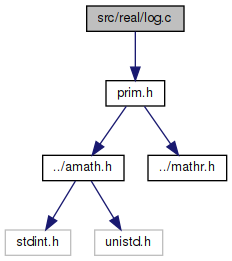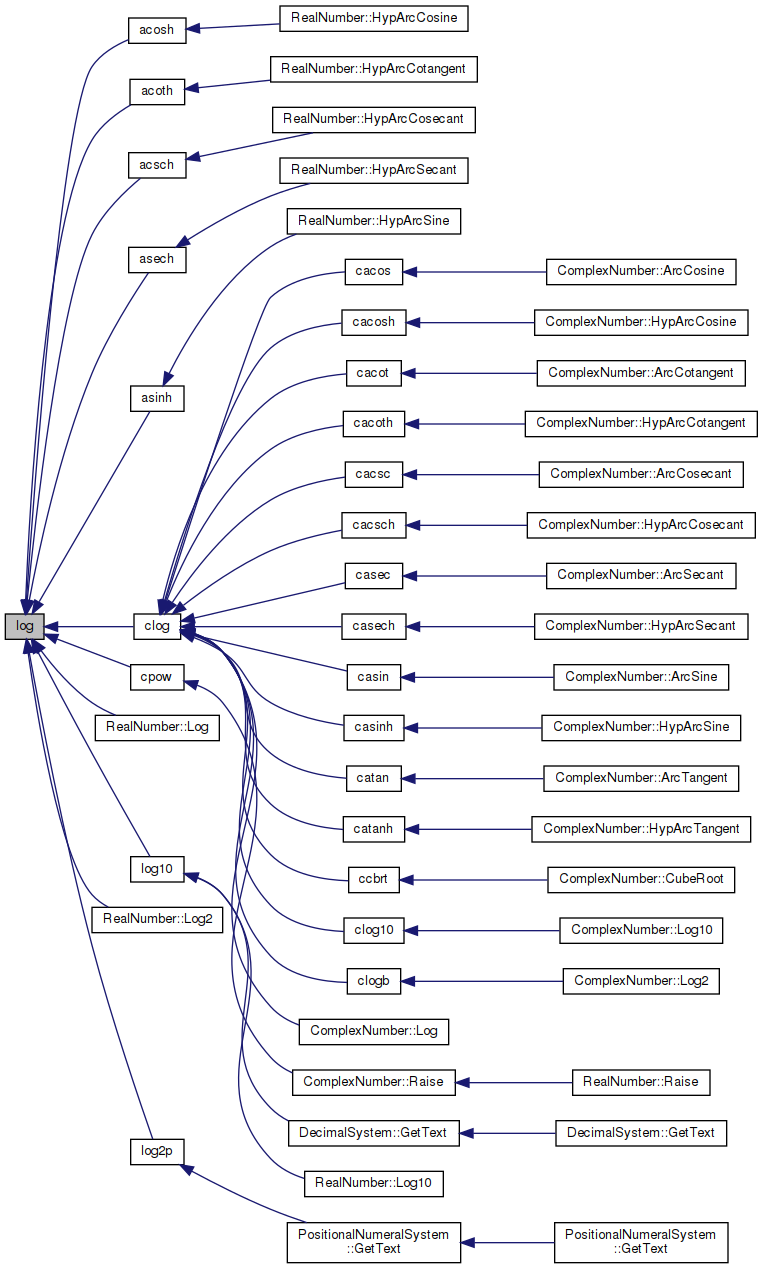amath  1.8.5 Simple command line calculator
log.c File Reference
`#include "prim.h"`
Include dependency graph for log.c:Go to the source code of this file.

## Functions

double log (double x)
Natural logarithm function (base e) More...

## Variables

static const double ln2_hi = 6.93147180369123816490e-01

static const double ln2_lo = 1.90821492927058770002e-10

static const double two54 = 1.80143985094819840000e+16

static const double Lg1 = 6.666666666666735130e-01

static const double Lg2 = 3.999999999940941908e-01

static const double Lg3 = 2.857142874366239149e-01

static const double Lg4 = 2.222219843214978396e-01

static const double Lg5 = 1.818357216161805012e-01

static const double Lg6 = 1.531383769920937332e-01

static const double Lg7 = 1.479819860511658591e-01

static double zero = 0.0

## ◆ log()

 double log ( double x )

Natural logarithm function (base e)

```Method
1. Argument Reduction: find k and f such that
x = 2^k * (1+f),
where  sqrt(2)/2 < 1+f < sqrt(2) .```
```  2. Approximation of log(1+f).
Let s = f/(2+f) ; based on log(1+f) = log(1+s) - log(1-s)
= 2s + 2/3 s**3 + 2/5 s**5 + .....,
= 2s + s*R
We use a special Remes algorithm on [0,0.1716] to generate
a polynomial of degree 14 to approximate R The maximum error
of this polynomial approximation is bounded by 2**-58.45. In
other words,
2      4      6      8      10      12      14
R(z) ~ Lg1*s +Lg2*s +Lg3*s +Lg4*s +Lg5*s  +Lg6*s  +Lg7*s
(the values of Lg1 to Lg7 are listed in the program)
and
|      2          14          |     -58.45
| Lg1*s +...+Lg7*s    -  R(z) | <= 2
|                             |
Note that 2s = f - s*f = f - hfsq + s*hfsq, where hfsq = f*f/2.
In order to guarantee error in log below 1ulp, we compute log
by
log(1+f) = f - s*(f - R)    (if f is not too large)
log(1+f) = f - (hfsq - s*(hfsq+R)). (better accuracy)```
``` 3. Finally,  log(x) = k*ln2 + log(1+f).
= k*ln2_hi+(f-(hfsq-(s*(hfsq+R)+k*ln2_lo)))
Here ln2 is split into two floating point number:
ln2_hi + ln2_lo,
where n*ln2_hi is always exact for |n| < 2000.```
```Special cases:
log(x) is NaN with signal if x < 0 (including -INF) ;
log(+INF) is +INF; log(0) is -INF with signal;
log(NaN) is that NaN with no signal.```
```Accuracy:
according to an error analysis, the error is always less than
1 ulp (unit in the last place).```
```Constants:
The hexadecimal values are the intended ones for the following
constants. The decimal values may be used, provided that the
compiler will convert from decimal to binary accurately enough
to produce the hexadecimal values shown.
```

Definition at line 109 of file log.c.

References Lg1, Lg2, Lg3, Lg4, Lg5, Lg6, Lg7, ln2_hi, ln2_lo, two54, and zero.

Referenced by acosh(), acoth(), acsch(), asech(), asinh(), clog(), cpow(), RealNumber::Log(), log10(), RealNumber::Log2(), and log2p().

110 {
111  double hfsq,f,s,z,R,w,t1,t2,dk;
112  int32_t k,hx,i,j;
113  uint32_t lx;
114
115  EXTRACT_WORDS(hx,lx,x);
116
117  k=0;
118  if (hx < 0x00100000) { /* x < 2**-1022 */
119  if (((hx&0x7fffffff)|lx)==0)
120  return -two54/zero; /* log(+-0)=-inf */
121  if (hx<0) return (x-x)/zero; /* log(-#) = NaN */
122  k -= 54;
123  x *= two54; /* subnormal number, scale up x */
124  GET_HIGH_WORD(hx,x); /* high word of x */
125  }
126  if (hx >= 0x7ff00000) return x+x;
127  k += (hx>>20)-1023;
128  hx &= 0x000fffff;
129  i = (hx+0x95f64)&0x100000;
130  SET_HIGH_WORD(x,hx|(i^0x3ff00000)); /* normalize x or x/2 */
131  k += (i>>20);
132  f = x-1.0;
133  if((0x000fffff&(2+hx))<3) { /* |f| < 2**-20 */
134  if(f==zero) {
135  if(k==0)
136  return zero;
137  else {
138  dk=(double)k;
139  return dk*ln2_hi+dk*ln2_lo;
140  }
141  }
142  R = f*f*(0.5-0.33333333333333333*f);
143  if(k==0) return f-R;
144  else {
145  dk=(double)k;
146  return dk*ln2_hi-((R-dk*ln2_lo)-f);
147  }
148  }
149  s = f/(2.0+f);
150  dk = (double)k;
151  z = s*s;
152  i = hx-0x6147a;
153  w = z*z;
154  j = 0x6b851-hx;
155  t1= w*(Lg2+w*(Lg4+w*Lg6));
156  t2= z*(Lg1+w*(Lg3+w*(Lg5+w*Lg7)));
157  i |= j;
158  R = t2+t1;
159  if(i>0) {
160  hfsq=0.5*f*f;
161  if(k==0) return f-(hfsq-s*(hfsq+R));
162  else
163  return dk*ln2_hi-((hfsq-(s*(hfsq+R)+dk*ln2_lo))-f);
164  } else {
165  if(k==0) return f-s*(f-R);
166  else
167  return dk*ln2_hi-((s*(f-R)-dk*ln2_lo)-f);
168  }
169 }
static const double Lg7
Definition: log.c:53
static const double Lg6
Definition: log.c:52
#define GET_HIGH_WORD(i, d)
Get the more significant 32 bit int from a double.
Definition: prim.h:167
static const double two54
Definition: log.c:46
static const double Lg2
Definition: log.c:48
#define EXTRACT_WORDS(ix0, ix1, d)
Get two 32 bit ints from a double.
Definition: prim.h:156
static double zero
Definition: log.c:55
static const double Lg3
Definition: log.c:49
static const double ln2_hi
Definition: log.c:44
static const double Lg4
Definition: log.c:50
static const double Lg1
Definition: log.c:47
#define SET_HIGH_WORD(d, v)
Set the more significant 32 bits of a double from an int.
Definition: prim.h:198
static const double ln2_lo
Definition: log.c:45
static const double Lg5
Definition: log.c:51
Here is the caller graph for this function:## ◆ Lg1

 const double Lg1 = 6.666666666666735130e-01
static

Definition at line 47 of file log.c.

Referenced by log().

## ◆ Lg2

 const double Lg2 = 3.999999999940941908e-01
static

Definition at line 48 of file log.c.

Referenced by log().

## ◆ Lg3

 const double Lg3 = 2.857142874366239149e-01
static

Definition at line 49 of file log.c.

Referenced by log().

## ◆ Lg4

 const double Lg4 = 2.222219843214978396e-01
static

Definition at line 50 of file log.c.

Referenced by log().

## ◆ Lg5

 const double Lg5 = 1.818357216161805012e-01
static

Definition at line 51 of file log.c.

Referenced by log().

## ◆ Lg6

 const double Lg6 = 1.531383769920937332e-01
static

Definition at line 52 of file log.c.

Referenced by log().

## ◆ Lg7

 const double Lg7 = 1.479819860511658591e-01
static

Definition at line 53 of file log.c.

Referenced by log().

## ◆ ln2_hi

 const double ln2_hi = 6.93147180369123816490e-01
static

Definition at line 44 of file log.c.

Referenced by log().

## ◆ ln2_lo

 const double ln2_lo = 1.90821492927058770002e-10
static

Definition at line 45 of file log.c.

Referenced by log().

## ◆ two54

 const double two54 = 1.80143985094819840000e+16
static

Definition at line 46 of file log.c.

Referenced by log().

## ◆ zero

 double zero = 0.0
static

Definition at line 55 of file log.c.

Referenced by log().